JEE  >  BITSAT Physics Test - 1

# BITSAT Physics Test - 1

Test Description

## 40 Questions MCQ Test BITSAT Mock Tests Series & Past Year Papers | BITSAT Physics Test - 1

BITSAT Physics Test - 1 for JEE 2023 is part of BITSAT Mock Tests Series & Past Year Papers preparation. The BITSAT Physics Test - 1 questions and answers have been prepared according to the JEE exam syllabus.The BITSAT Physics Test - 1 MCQs are made for JEE 2023 Exam. Find important definitions, questions, notes, meanings, examples, exercises, MCQs and online tests for BITSAT Physics Test - 1 below.
Solutions of BITSAT Physics Test - 1 questions in English are available as part of our BITSAT Mock Tests Series & Past Year Papers for JEE & BITSAT Physics Test - 1 solutions in Hindi for BITSAT Mock Tests Series & Past Year Papers course. Download more important topics, notes, lectures and mock test series for JEE Exam by signing up for free. Attempt BITSAT Physics Test - 1 | 40 questions in 50 minutes | Mock test for JEE preparation | Free important questions MCQ to study BITSAT Mock Tests Series & Past Year Papers for JEE Exam | Download free PDF with solutions
 1 Crore+ students have signed up on EduRev. Have you?
BITSAT Physics Test - 1 - Question 1

### In LCR circuit, the capacitance is changed from C to 4C. For the same resonant frequency the inductance should be changed from L to

Detailed Solution for BITSAT Physics Test - 1 - Question 1

For resonance:
XL ​= XC​
ωL = 1/ωC
ω = 1/√2C​​
2πf = 1/√2C​
For same resonant frequency:
LC should be constant.
So, LC = 4CL1​
⇒ L1 = LC/4C​ = L/4​

BITSAT Physics Test - 1 - Question 2

### The mass number of a nucleus is

Detailed Solution for BITSAT Physics Test - 1 - Question 2

A = N + Z, where A is mass number and Z is atomic number and N is number of neutrons
So A > Z except for H-atom in which A = Z as N = 0

BITSAT Physics Test - 1 - Question 3

### The radius of the first orbit of the hydrogen atom is a₀. The radius of the second orbit will be

Detailed Solution for BITSAT Physics Test - 1 - Question 3

The radius of the first bohr orbit = a0​ According to 3rd Postulate of bohr atomic model

L = mvr = rh/2π​; where n=1,2,3

⇒ For nth orbit
r​= a0​n2
Radius of second orbit = a0(2)2 = 4a0

BITSAT Physics Test - 1 - Question 4

If rigidity modulus is 2.6 times of youngs modulus then the value of poission's ratio is

Detailed Solution for BITSAT Physics Test - 1 - Question 4

Young’s Modulus = 2 × Rigidity Modulus × (1+ poisson’s Ratio)
Given, Young’s Modulus = 2.6 × Rigidity Modulus
2.6 = 2×(1+σ)
1.3 = 1 + σ
σ = 0.3​

BITSAT Physics Test - 1 - Question 5

Cathode rays are similar to visible light rays in that

Detailed Solution for BITSAT Physics Test - 1 - Question 5

Both the cathode rays and the visible light rays (because of having particle nature) affect the photographic plates when incident on it. So, cathode rays and visible light rays similar in that sense.

BITSAT Physics Test - 1 - Question 6

The energy of a photon of wavelength (λ) is

Detailed Solution for BITSAT Physics Test - 1 - Question 6

The energy of a photon is directly proportional to its frequency with proportionality constant being the Plank's constant h.

Hence E = hν = hc/λ​  (Since c=λν)

⟹ E = hc/λ

BITSAT Physics Test - 1 - Question 7

By increasing the temperature, the specific resistance of a conductor and a semiconductor

Detailed Solution for BITSAT Physics Test - 1 - Question 7
• Because specific resistance depends upon value of α the temperature coefficient of the resistance. the value α for conductors is positive and for semiconductors the value of α is negative.
• Hence By increasing the temperature, the specific resistance of a conductor increases and that of a semiconductor decreases.
BITSAT Physics Test - 1 - Question 8

The power factor of a series LCR circuit when at resonance is

Detailed Solution for BITSAT Physics Test - 1 - Question 8

At resonance, the impedance of the circuit is equal to the resistance in the circuit, i.e. Z = R
∴ power factor of the circuit, cos φ = R/Z = R/R = 1

BITSAT Physics Test - 1 - Question 9

Minimum no. of 8 μF and 250 V capacitors, used to make a combination of 16 μF and 1000 V, is

Detailed Solution for BITSAT Physics Test - 1 - Question 9

To have 1000 V tolerance, you need to stack up four 8μF capacitor in series. The equivalent capacitance of 4 such capacitors is:
⟹ Ceq​ = (8μF)/4​ = 2μF
To make a total of 16 μF that tolerates 1000V, you need 8 set of them arranged in parallel such that
⟹Ceq′​ = 8 × 2μF = 16μF
∴ Total number of capacitors = 8×4 = 32

BITSAT Physics Test - 1 - Question 10

A fireman of mass 60 kg slides down a pole. He is pressing the pole with a force of 600 N. The coefficient of friction between the hands and the pole is 0.5, with what acceleration will the fireman slide down (g=10m/s2)

Detailed Solution for BITSAT Physics Test - 1 - Question 10

Here mass, m = 60kg

and coefficient of friction, μ = 0.5

and g =10m/s2

Force applied by fireman, F = 600N

Friction Force, f = μmg = 0.5×60×10 = 300N

Net movement force,

F − f = ma
⟹ 600 − 300 = ma
⟹ a = 300/60​
⟹ a = 5m/s2

Hence the acceleration with which the fireman slide down is 5m/s2

BITSAT Physics Test - 1 - Question 11

Orbit of a planet around a star is

Detailed Solution for BITSAT Physics Test - 1 - Question 11

It is evident from Kepler’s first law of planetary motion that “The orbit of a planet is an ellipse with the Sun at one of the two foci.”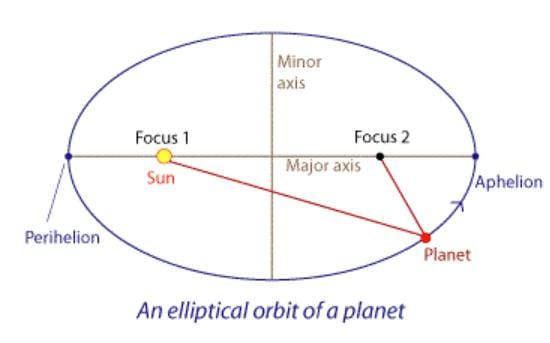BITSAT Physics Test - 1 - Question 12

A body takes T minutes to cool from 62 degree C to 61 degree C when the surrounding temperature is 30 degree C. The time taken by the body to cool from 46 degree C to 45.5 degree C is

Detailed Solution for BITSAT Physics Test - 1 - Question 12

According to Newton's law of cooling,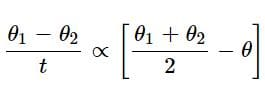For the first condition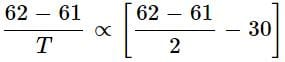..... (i)
and for the seconds condition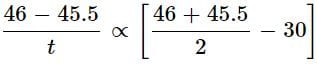...... (ii)
By soving Eqs. (i) and (ii) we get t = T minutes.

BITSAT Physics Test - 1 - Question 13

An ideal gas is heated from 27°C to 627°C at constant pressure. If initial volume was 4 m3, then the final volume of the gas will be

Detailed Solution for BITSAT Physics Test - 1 - Question 13

Given, Initial temperature (T1) : 27°C = 300 K
Final temperature (T2) = 621°C = 900 K

Initial volume (V1) = 4 m3.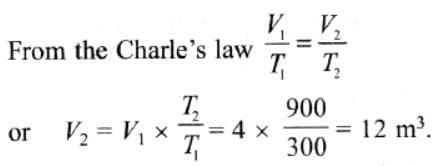BITSAT Physics Test - 1 - Question 14

The monoenergetic beams of electrons moving along + y direction enters a region of uniform electric and magnetic fields. If the beam goes straight through, then these simultaneously fields B̅ and E̅ are directed respectively

Detailed Solution for BITSAT Physics Test - 1 - Question 14

V = Vj​
Hence, B and E should be perpendicular to each other and also perpendicular to the velocity. Hence, B and E should be in z-axis and x-axis
If B is towards + z axis , Force = q(V \times B ) is in + x direction . To balance this , E has to be in -x direction.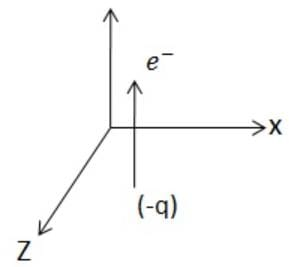BITSAT Physics Test - 1 - Question 15

If a substance is to be paramagnetic, its atoms must have a

Detailed Solution for BITSAT Physics Test - 1 - Question 15

Paramagnetic materials possess a permanent magnetic dipole moment due to incomplete cancellation of electron spin and orbital magnetic moment.

BITSAT Physics Test - 1 - Question 16

Horizontal tube of non-uniform cross-section has radii of 0.1m and 0.05m respectively at M and N. For a streamline flow of liquid the rate of liquid flow is

Detailed Solution for BITSAT Physics Test - 1 - Question 16

According to principle of continuity, for streamline flow of fluid through a tube of non-uniform cross-section the rate of flow of fluid (Q) is same at every point in the tube.
i.e, Av = constant
⇒  A1​v​= A2​v2
Therefore, the rate of flow of fluid is same at M and N.

BITSAT Physics Test - 1 - Question 17

The viscous drag on a spherical body moving with a speed ν in a viscous medium is directly proportional to

Detailed Solution for BITSAT Physics Test - 1 - Question 17

The viscous drag on a spherical body is given as F = 6πηRV.

It is directly proportional to V. Here η is the coefficient of viscosity, R is the radius of the sphere and V is its velocity.

BITSAT Physics Test - 1 - Question 18

The ratio of tangential stress to the shearing strain is called

Detailed Solution for BITSAT Physics Test - 1 - Question 18

The ratio of shearing stress to the corresponding shearing strain is called shear modulus or modulus of rigidity.

BITSAT Physics Test - 1 - Question 19

A particle of charge -q and mass m moves in a circle of radius r around an infinitely long line charge of linear charge density + λ . Then time period will be:(Where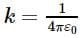)

BITSAT Physics Test - 1 - Question 20

The initial velocity of a body moving along a straight line is 7 m/s. It has a uniform acceleration of 4 m/s2. The distance covered by the body in the 5th second of its motion is

Detailed Solution for BITSAT Physics Test - 1 - Question 20

Given, u = 7m/s

a = 4m/s2

n = 5

The distance covered by the body in nth sec is given by

Sn​ = u + a/2​(2n−1)

S5​ = 7 + 24​(2×5−1)

S5​ = 25 m

BITSAT Physics Test - 1 - Question 21

A particle is moving in a circle with uniform speed υ. In moving from a point to another diametrically opposite point

Detailed Solution for BITSAT Physics Test - 1 - Question 21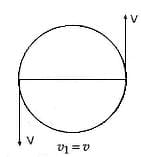Initial velocity v1 = v
Final velocity v2 = -v
Initial momentum p= mv
Final momentum p= m(-v) = -mv
Change in momentum
Δp = p1 - p2
⇒ mv - (-mv)
⇒ 2mv

BITSAT Physics Test - 1 - Question 22

If a particle of mass m is moving in a horizontal circle of radius r with a centripetal force (-k/r2), the total energy is

Detailed Solution for BITSAT Physics Test - 1 - Question 22

Given, Centripetal force = mv2​/r = -k/r2
Kinetic Energy =​ mv2/2 = k/2r
Potential Energy = −∫r∞ ​Fdr
the lower limit has been taken as ∞ because potential energy is zero at infinty

⇒ −∫r∞ (k/r2)​dr

⇒ −k ∫r∞ ​r−2dr

⇒ −k |r-1/-1|r

⇒ −k/r​

Total energy = k/2r​ − k/r ​= −k/2r​

BITSAT Physics Test - 1 - Question 23

A body of mass 2 kg has an initial velocity of 3 metres per second along OE and it is subjected to a force of 4 N in a direction perpendicular to OE. The distance of the body from O after 4 seconds will be

Detailed Solution for BITSAT Physics Test - 1 - Question 23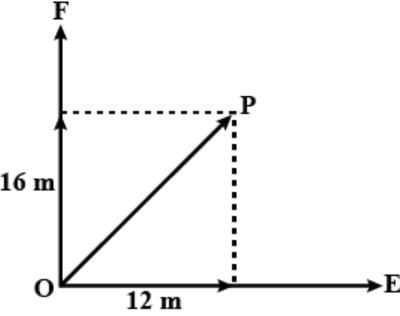Along OE​

Velocity, VOE​=3 m/s

Since, applied force is perpendicular to OE so the velocity VOE​ will be constant.

So, displacement along OE in 4 sec SOE​=3×4=12 m

Along OF​

Force applied, F = 4 N

Mass of the body, m = 2 kg

So, acceleration a = mF ​= 2 m/s2

Displacement along OF in time t = 4 sec

SOF​ = ut + ​at2/2

SOF ​= 0 + 2×42/2

SOF​ = 16 m

After t = 4 sec, the distance of the body from O to OP

OP= 12+ 162

OP2 = 144 + 256

OP2 = 400

OP = 20 m

BITSAT Physics Test - 1 - Question 24

An astronomical telescope has objective and eye-piece lens of powers 0.5 D and 20 D respectively. Its magnifying power will be

Detailed Solution for BITSAT Physics Test - 1 - Question 24

Given, the power of objective lens,
Po​ = 0.5D
The power of eye-piece lens,
P​= 20D
The magnifying power of an astronomical telescope
M = fo​​/fe
or = ​Pe/Po​​    [∴ P = 1/f​]
= 20/0.5
= 40

BITSAT Physics Test - 1 - Question 25

A simple pendulum executing simple harmonic motion is falling freely along with the support. Then

Detailed Solution for BITSAT Physics Test - 1 - Question 25

Time period of a simple pendulum,
T = (2π√l)/√g​​
So, T = ∞
∴ pendulum does not oscillate at all.

BITSAT Physics Test - 1 - Question 26

In the absence of damping, the amplitude of forced oscillation at resonance is

Detailed Solution for BITSAT Physics Test - 1 - Question 26

When there is no damping , the damping constant b = 0 and at resonace, the amplitude of forced oscillations is infinite.

BITSAT Physics Test - 1 - Question 27

The moment of inertia of a cylinder of radius R, length l and mass M about an axis passing through its centre of mass and normal to its length, is

Detailed Solution for BITSAT Physics Test - 1 - Question 27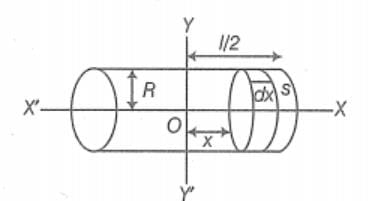Let, XX' be the axis of symmetry and YY' be the axis perpendicular to XX'.

Let us consider a circular disc S of width dx at a distance x from YY' axis.

Mass per unit length of the cylinder is M​/l.

Thus, the mass of disc is M​dx/l
Moment of inertia of this disc about the diameter of the rod = (M​dx/l)R2/4​.
Moment of inertia of disc about YY' axis given by parallel axes theorem is = (M​dx/l)R2​/4 + (M​dx/l)x2
Moment of inertia of cylinder,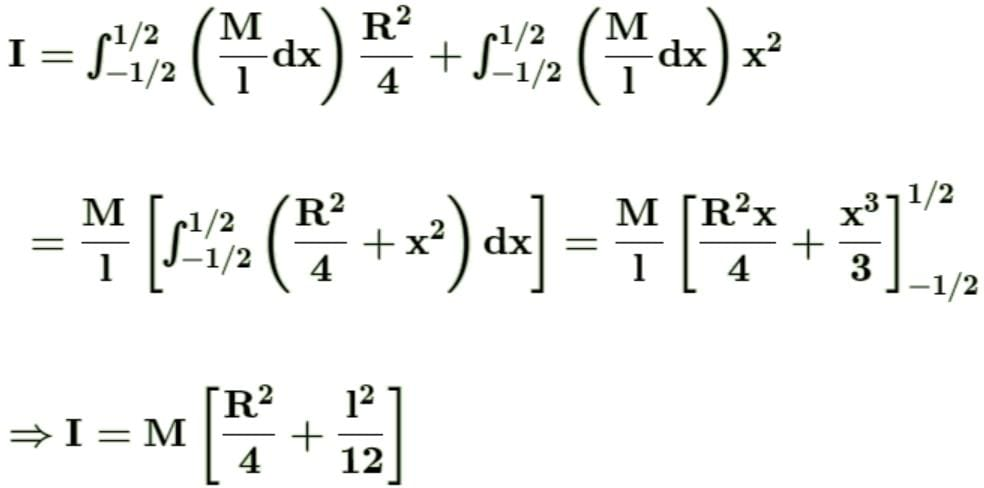BITSAT Physics Test - 1 - Question 28

Two bodies of different masses of 2 kg and 4 kg are moving with velocities 2 m/s and 10 m/s towards each other due to mutual gravitational attraction. What is velocity of their centre of mass ?

Detailed Solution for BITSAT Physics Test - 1 - Question 28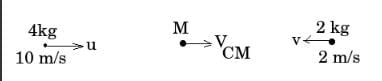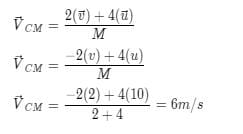BITSAT Physics Test - 1 - Question 29

The radius of curvature of a thin plano-convex lens is 10 cm (of curved surface) and the refractive index is 1.5. If the plane surface is silvered, then the focal length will be

Detailed Solution for BITSAT Physics Test - 1 - Question 29

The focal length of a combination of lenses, is given by,
f = R​/2(μ−1)
Substituting, the values R = −10 cm and μ = 1.5
We get, f = −10 cm
Here, -ve sign shows that it will behave like a concave mirror.

BITSAT Physics Test - 1 - Question 30

A force of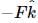acts on O, the origin of the coordinate system. The torque about the point (1, -1) is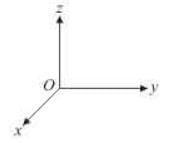Detailed Solution for BITSAT Physics Test - 1 - Question 30

Torque = r x F

r = î - j

so torque = (i - j) x -Fk

= -F(-j - i)

= F( i + j)

BITSAT Physics Test - 1 - Question 31

A bulb rated at (100W-200V) is used on a 100V line. The current in the bulb is

Detailed Solution for BITSAT Physics Test - 1 - Question 31

We know that P = V2 / R
Thus as P = 100W and V = 200V we get R = 40000/100 = 400Ω
Now as we know V = IR
We get I = V / R
= 100 / 400
= ¼ A

BITSAT Physics Test - 1 - Question 32

A monoatomic gas is suddenly compressed to 1/8 of its volume adiabatically. The ratio of pressure of the gas now to that of its original pressure is
(Given, the ratio of the specific heats of the given gas to be 5/3)

Detailed Solution for BITSAT Physics Test - 1 - Question 32

It is given that Cp/Cv = γ = 5/3​

P1​V1γ​ = P2​V2γ

⟹ ​P2/P1 ​​= (​V1/V2​​)5/3

= (8​)5/3 = 32

BITSAT Physics Test - 1 - Question 33

5 mole of hydrogen gas is heated from 30oC to 60oC at constant pressure. Heat given to the gas is (given R = 2 cal/mole degree)

Detailed Solution for BITSAT Physics Test - 1 - Question 33

Hydrogen is diatomic.
Hence, molar heat capacity, Cp​ = 3.5R
Also change in temperature = ΔT = 30K, no.of moles, n = 5
Hence, heat absorbed = ΔQ = nCp​ΔT = 5×3.5×2×30 = 1050cal

BITSAT Physics Test - 1 - Question 34

Unit of moment of inertia in MKS system

Detailed Solution for BITSAT Physics Test - 1 - Question 34

We know that the moment of inertia of a body is given as:
l = MR2
So, the unit of moment of interia will be kg m2

BITSAT Physics Test - 1 - Question 35

Wave optics is based on wave theory of light put forward by Huygen and modified later by

Detailed Solution for BITSAT Physics Test - 1 - Question 35

The Huygens-Fresnel principle states that every point on a wavefront is a source of wavelets. These wavelets spread out in the forward direction, at the same speed as the source wave. The new wavefront is a line tangent to all of the wavelets.

BITSAT Physics Test - 1 - Question 36

Huygen's wave theory allows us to know

Detailed Solution for BITSAT Physics Test - 1 - Question 36

Huygen's wave theory explain the propagation of the wavefront.

BITSAT Physics Test - 1 - Question 37

If the equation of SHM is y = a sin (4 π t + φ) how much is its frequency?

Detailed Solution for BITSAT Physics Test - 1 - Question 37

Comparing with a standard Equation of SHM ,
y = a sin(wt + φ)

ω = 4π, and θ = φ = initial phase.

So frequency is as follows :
freq. = 4π/2π
⇒ f = 2 Hz

So final answer is 2 Hz frequency.

BITSAT Physics Test - 1 - Question 38

The phase difference between two points separated by 0.8 m in a wave of frequency 120 Hz is 90o. Then the velocity of wave will be

Detailed Solution for BITSAT Physics Test - 1 - Question 38

Δϕ = 90∘ = a​/2

Δx = 0.8m

we know that Δϕ = (2π/α).Δx

So λ = (2π.Δx)/Δϕ ​= (2π×0.8)/​(π/2)

⇒ λ = 3.2m​

v = 120 Hz & v = vλ

velocity v = 120×3.2 = 384m/s​

BITSAT Physics Test - 1 - Question 39

A solid disc of mass M is just held in air horizontally by throwing 40 stones per sec vertically upwards to strike the disc each with a velocity of 6 ms⁻1. If the mass of each stone os 0.05 kg what is the mass of the disc. (g = 10 ms⁻2)

Detailed Solution for BITSAT Physics Test - 1 - Question 39

Weight of the disc will be balanced by the force applied by the bullet on the disc in vertically upward direction
F = nmv = 40X0.05X6 = mg
M = (40x0.05x6)/16 = 1.2 kg

BITSAT Physics Test - 1 - Question 40

A particle of mass m moving with velocity ν collides with a stationary particle of mass 2 m and sticks to it. The speed of the system, after collision, will be

Detailed Solution for BITSAT Physics Test - 1 - Question 40

Mass of first particle is m1​ = m and its velocity before collision  u1​ = v

Mass of second particle is m2​ = 2m and its velocity before collision  u2 ​= 0

Let the velocity of combined system just after collision  be V.

Using conservation of linear momentum :

m1​u1​ + m2​u2 ​= (m1​ + m2​)V

∴  (m)(v) + (2m)(0) = (m + 2m)V

⟹  V = v/3​

## BITSAT Mock Tests Series & Past Year Papers

2 videos|15 docs|70 tests
 Use Code STAYHOME200 and get INR 200 additional OFF Use Coupon Code
Information about BITSAT Physics Test - 1 Page
In this test you can find the Exam questions for BITSAT Physics Test - 1 solved & explained in the simplest way possible. Besides giving Questions and answers for BITSAT Physics Test - 1, EduRev gives you an ample number of Online tests for practice

## BITSAT Mock Tests Series & Past Year Papers

2 videos|15 docs|70 tests